# Power allocation strategies for distributed precoded multicell based systems

## Abstract

Multicell cooperation is a promising solution for cellular wireless systems to mitigate intercell interference, improve system fairness, and increase capacity. In this article, we propose power allocation techniques for the downlink of distributed, precoded, multicell cellular-based systems. The precoder is designed in two phases: first the intercell interference is removed by applying a set of distributed precoding vectors; then the system is further optimized through power allocation. Three centralized power allocation algorithms with per-BS power constraint and different complexity trade-offs are proposed: one optimal in terms of minimization of the instantaneous average bit error rate (BER), and two suboptimal. In this latter approach, the powers are computed in two phases. First, the powers are derived under total power constraint (TPC) and two criterions are considered, namely, minimization of the instantaneous average BER and minimization of the sum of inverse of signal-to-noise ratio. Then, the final powers are computed to satisfy the individual per-BS power constraint. The performance of the proposed schemes is evaluated, considering typical pedestrian scenarios based on LTE specifications. The numerical results show that the proposed suboptimal schemes achieve a performance very close to the optimal but with lower computational complexity. Moreover, the performance of the proposed per-BS precoding schemes is close to the one obtained considering TPC over a supercell.

## Introduction

Multiple input and multiple output (MIMO) is a very promising technique to mitigate the channel fading and thus improving the cellular system capacity . On the other hand, orthogonal frequency division multiplexing (OFDM) is a simple technique to mitigate the effects of inter-symbol interference in frequency selective channels. Combining OFDM with MIMO, producing the so-called MIMO-OFDM, significantly reduces receiver complexity in wireless multiuser broadband systems, thus making it a competitive choice for future broadband wireless communication systems [2, 3].

The conventional cellular architecture considers co-located MIMO and single cell-processing techniques. However, the problems inherent of these systems, such as shadowing, significant correlation between channels in some environments, and intercell interference, significantly degrades the capacity gains promised by MIMO techniques . Thus, in a multicell environment to fully exploit the multiple antenna gain, base station (BS) cooperation is required. Such systems have the advantage of macro-diversity that is inherent to the widely spaced antennas and more flexibility to deal with intercell interference, which fundamentally limits the performance of user terminals (UTs) at cell edges [5, 6]. An enhanced cellular architecture is being proposed and implemented, under the European FUTON project [7, 8].

In recent years, relevant works on multicell precoding techniques have been proposed in . The multicell downlink channel is closely related to the MIMO broadcast channel, where the optimal precoding is achieved by the dirty paper coding (DPC) principle . However, the significant amount of processing complexity required by DPC prohibits its implementation in practical multicell processing. Some suboptimal multicell linear precoding schemes have been discussed in , where analytic performance expressions for each scheme were derived considering nonfading scenario with random phases. The comparison of the achievable rates by the different proposed cooperative schemes showed a trade-off between performance improvement and the requirement for BS cooperation, signal-processing complexity, and channel state information (CSI) knowledge. In , the impact of joint multicell site processing was discussed through a simple analytically tractable circular multicell model. The potential improvement in downlink throughput of cellular systems using limited network coordination to mitigate intercell interference has been discussed in , where zero forcing (ZF) and DPC-precoding techniques under distributed and centralized architectures have been studied. In [12, 13], a clustered BS coordination is enabled through a multicell block diagonalization (BD) scheme to mitigate the effects of interference in multicell MIMO systems. Three different power allocation algorithms were proposed with different constraints to maximize the sum rate. A centralized precoder design and power allocation was considered. In , the inner bounds on capacity regions for downlink transmission were derived with or without BS cooperation and under per-antenna power or sum-power constraint. Those authors showed that under imperfect CSI, significant gains are achievable by BS cooperation using linear precoding. Furthermore, the type of cooperation depends on channel conditions to optimize the rate/backhaul trade-off. Two multicell precoding schemes based on the water-filling technique have been proposed in . It was shown that these techniques achieve a performance, in terms of weighted sum rate, very close to the optimal. In , each BS performs ZF locally to remove the channel interference and based on the statistical knowledge of the channels, the central unit (CU) performs a centralized power allocation that jointly minimizes the outage probability of the UTs. A decentralized multicell cooperative processing framework has been proposed in . In the proposed scheme, each UT feeds back its CSI to all collaborative BSs, and the needed operations of user scheduling and signal processing are performed in a distributed fashion. A new BD cooperative multicell scheme has been proposed in , to maximize the weighted sum-rate achievable for all the UTs. Multiuser multicell precoding with distributed power allocation has been discussed in . It is assumed that each BS has only the knowledge of local CSI, and based on that the beamforming vectors used to achieve the outer boundary of the achievable rate region were derived considering both instantaneous and statistical CSI.

In this article, we design and evaluate power allocation techniques for precoded multicell-based systems. The precoder design has two aims: allow spatial users separation and optimize the power allocation. The two problems can be decoupled leading to a two-step design: the distributed precoder vector design, and three centralized power allocation algorithms with different complexities. To design the precoder vector, we assume that BSs have only knowledge of local CSI, reducing the feedback load over the backhaul network. Then, the system is further optimized by proposing three centralized power allocation algorithms with per-BS power constraint: one optimal to minimize the average bit error rate (BER), for which the powers can be obtained numerically by using convex optimization, and two suboptimal. In this latter approach, the powers are computed in two phases. First the powers are derived under total power constraint (TPC). Two criterions are considered, namely minimization of the average BER, which leads to an iterative approach and minimization of the sum of inverse of signal-to-noise ratio for which closed form solution is achieved. Then, the final powers are computed to satisfy the individual per-BS power constraint. Since we assume a centralized power allocation, some channel information must be shared among all BSs in each supercell, even considering that the precoder vectors are computed in a distributed manner on each BS.

The remainder of the article is organized as follows: Section "System model" presents the system model. Section "Multicell precoding schemes" describes the proposed multiuser multicell precoding techniques with distributed precoder design and centralized power allocation. Section "Numerical results" presents the main simulation results. Finally, the conclusions will be drawn.

## System model

Throughout this article, we will use the following notations. Lowercase letters, boldface lowercase letters, and boldface uppercase letters are used for scalars, vectors, and matrices, respectively.

(.)H, (.)T, (.)* represent the conjugate transpose, the transpose, and complex conjugate operators, respectively. E{.} represents the expectation operator, I N is the identity matrix of size N × N,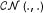denotes a circular symmetric complex Gaussian vector, and [A] i is the i th column of the matrix A.

Multicell architectures that assume a global coordination can eliminate the intercell interference completely. However, in practical cellular scenarios, issues such as the complexity of joint signal processing of all the BSs, the difficulty in acquiring full CSI from all UTs at each BS, and synchronization requirements will make global coordination difficult. Therefore, we assume a clustered multicell cellular system as shown in Figure 1, where the base stations are linked to a CU (e.g., by optical fiber) as proposed in [7, 8]. In such architecture the area covered by the set of cooperating BSs is termed as supercell. The area defined by all the supercells that are linked to the same CU is termed as serving area. The BSs corresponding to a supercell are processed jointly by a joint processing unit (JPU). The number of cooperating BSs per supercell should not be high for the reasons discussed above. In this study, it is assumed that the interference between the supercells is negligible. In fact, as we are replacing the concept of cell by the one of supercell, this means that there will be some interference among the supercells especially at the edges. Two approaches can be considered to deal with the inter-supercell interference. The precoders are designed to remove both intra-supercell and inter-supercell interferences, but as discussed in , this strategy reduces the number of degrees of freedom to efficiently eliminate the intra-supercell interference. Alternatively, the radio resource management can be jointly performed for a large set of supercells (the serving area) at the CU, and thus the resource allocation can be done in a way that the UTs of each supercell edge interfere as little as possible with the users of other supercells , justifying our assumption to neglect it. This resource allocation problem is, however, beyond the scope of this article. In this latter approach, all degrees of freedom can be used to efficiently eliminate the intra-supercell interference.

For the sake of simplicity, in this analysis, we start by assuming a narrow band transmission, since the results can be easily extended for multicarrier-based systems as discussed later. In this article, we consider B-distributed BSs per supercell, with each BS being equipped with N tb antennas, transmitting to K UTs as shown in Figure 2. The total number of transmitting antennas is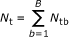. The UTs are equipped with a single antenna, and we assume that NtbKa.

Under the assumption of linear precoding, the signal transmitted by the BS b is given by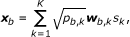(1)

where p b,k represents the power allocated to UT k on BS b, and w b,k Ntbx 1is the precoder of user k at BS b with unit norms, i.e., ||w b,k || = 1, b = 1, ..., B, k = 1, ..., K. The data symbol s k , with E{|s k |2} = 1, is intended for UT k and is assumed to be available at all BSs of the same supercell. The average power transmitted by the BS b is then given by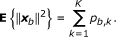(2)

The received signal at the UT k, y k 1x 1, can be expressed by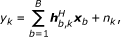(3)

where h b,k Ntbx 1represents the flat fading channel between BS b and UT k and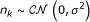the noise.

The channel h b,k can be decomposed as the product of the fast fading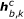and slow fading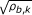components, i.e.,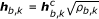, where ρ b,k represents the long-term power gain between BS b and user k. The Ntb fast fading components may exhibit correlation, and we further decompose it as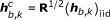, where (h b,k )iid contains the fast fading coefficients with iid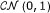entries and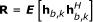is the normalized transmitter correlation matrix defined in , which is assumed to be the same for all BSs. The antenna channels from BS b to user k, i.e., the components of, may be correlated, but the links seen from different BSs to a given UT are assumed to be uncorrelated as the BSs of one supercell are geographically separated.

## Multicell-precoding schemes

The multicell-precoding schemes are considered in two phases: the distributed precoder vectors and centralized power allocation design.

### Distributed precoder vectors

To design the precoder vectors, we assume that the BSs have only knowledge of local CSI, i.e., BS b knows the instantaneous channel vectors h b,k , k = 1, ..., K. We consider a ZF transmission scheme with the phase of the received signal at each UT aligned. From Equations 1 and 3, the received signal at UT k can be decomposed in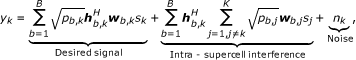(4)

where w b,k is a unit-norm ZF vector orthogonal to K - 1 channel vectors,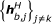. Such precoding vectors always exist because we assume that the number of antennas at each BS is higher or equal to the number of single antenna UTs, i.e., NtbK. By using such precoding vectors, the intra-supercell interference is canceled and each data symbol is only transmitted to its intended UT. Also, as discussed in  for any precoding vector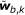in the null space of,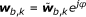is also in the null space of. Thus, we can choose the precoding vectors such that the terms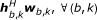all have zeros phases, i.e.,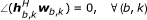. These precoding vectors can be easily computed, and so if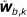is found to lie in the null space of, the final precoding vector, with the phase of the received signal at each UT aligned, is given by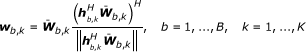(5)

where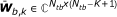holds the (Ntb - K + 1) singular vectors in the null space of. For the case where Ntb = K, only one vector lies in the null space of, but for Ntb > K, more than one vector lie in the null space of. In this latter case, the final vector w b,k is a linear combination of the (Ntb - K + 1) possible solutions. The equivalent channel between BS b and UT k can be expressed as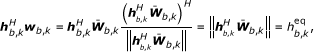(6)

and from Equation 6, we can observe that the equivalent channel,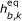, is a positive real number. By using the precoding vectors defined in Equation 5 and considering Equation 6, the received signal in Equation 4 reduces to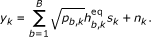(7)

It should be mentioned that at the UT, to allow high order modulations, only the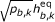coefficients are needed to be estimated instead of all the complex coefficients of the channel, leading to a UT design of low complexity. As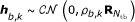and the columns of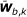have unit norm and are independent of h b,k , the (Ntb - K + 1) components of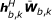are iid Gaussian variables with varianceper component. The variance δ b,k depends on the correlation matrix R. When R = INtb, i.e., uncorrelated links seen by a single BS to each UT,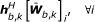is a linear combination of iid complex Gaussian variables, and thus δ b,k = ρ b,k , (b, k). When, R = 1Ntbthe elements of h b,k are fully correlated, and thus the sum of the elements of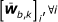is zero, and then δ b,k = 0, (b, k).

Since the (Ntb - K + 1) components ofare iid Gaussian variables,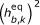is a chi-square random variable with 2(Ntb - K + 1) degrees of freedom. Once thevariables are independent, each user is expected to achieve a diversity order of B(Ntb - K + 1) (assuming that all channels have the same average power, i.e., ρb,k = ρ, (b,k) and p b,k = 1, (b,k)). Also, because the received signals from different BSs have the same phase, they are added coherently at the UTs, and thus an additional antenna gain is achieved.

### Power allocation strategies

In this section, one optimal power strategy, to minimize the average BER, and two suboptimal ones are considered. Our motivation to minimize the BER is that, from the practical point of view, a system that minimizes the BER is preferable rather than the one that maximizes the sum capacity. Maximization of the capacity provides the upper bound on the achievable sum rate, but this is only approached if one is able to find the optimum processing. In fact, most of the studies on multicell aiming to maximize the capacity (e.g., ), assume Gaussian signals at the input of the channel, which is not realistic in practical systems, where signals from discrete constellations are used . Thus, using the BER as criteria, we are closer to realizability while capacity only ensures achievability. The aim is to design practical multicell power allocation techniques to improve the user's fairness, namely at the cell-edges inside each supercell.

### Optimal minimum BER power allocation

From Equation 7 the instantaneous SNR of user k can be written as(8)

and assuming a M-ary QAM constellations, the instantaneous probability of error for user k is given by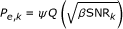(9)

where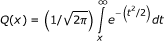, β = 3/(M - 1), and.

We minimize the instantaneous average probability under the per-BS power constraint Ptb, i.e.,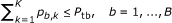. Without loss of generality, we assume a 4-QAM constellation, and thus the optimal power allocation problem with per-BS power constraint can be formulated as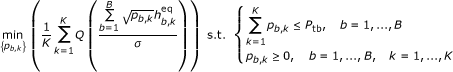(10)

Since the objective function is convex in p b,k , and the constraint functions are linear, this is a convex optimization problem. Therefore, it may be solved numerically by using, for example, the interior-point method . This scheme is referred as per-BS optimal power allocation (per-BS OPA).

### Sub-OPA approaches

Since the complexity of the above scheme is too high, and thus it is not of interest for real wireless systems, we also resort to less complex suboptimal solutions. The proposed strategy has two phases: first the power allocation is computed by assuming that all BSs of each supercell can jointly pool their power, i.e., a TPC Pt is imposed instead and the above optimization problem reduces to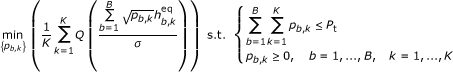(11)

with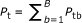and using the Lagrange multipliers method , the following cost function with μ Lagrange multiplier is minimized: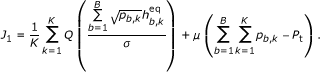(12)

The powers p b,k , (b,k) can be determined by setting the partial derivatives of J1 to zero and as shown in appendix the solution is(13)

where W0 stands for Lambert's W function of index 0 . This function W0(x) is an increasing function with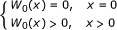. Therefore, μ2 can be determined iteratively, using constraint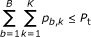. One solution based on Lambert W function that minimizes the instantaneous BER was also derived in the context of single user single cell MIMO systems .

The optimization problem of Equation 11 is similar to the single cell power allocation optimization problem, where the users are allocated the same total multicell power, which may serve as a lower bound of the average BER for the multicell with per-BS power constraint.

The second phase consists of replacing μ2 by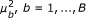in Equation 13, and then computing iteratively different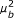to satisfy the individual per-BS power constraints instead, i.e.,are computed to satisfy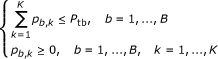. This suboptimal scheme is referred as per-BS sub-optimal iterative power allocation (per-BS SOIPA).

Although this suboptimal solution significantly reduces the complexity relatively to the optimal one, it still needs an iterative search. To further simplify, we propose an alternative power allocation method based on minimizing the sum of inverse of SNRs, for which closed-form expression can be obtained. Note that minimizing the sum of inverse of SNRs is similar to the maximization of the harmonic mean of the SINRs discussed in . In this case, the optimization problem is written as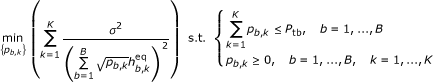(14)

Since the objective function is convex in p b,k , and the constraint functions are linear, Equation 14 is also a convex optimization problem. To solve it, we follow the same suboptimal two phases approach as for the first problem.

First, we impose a TPC and the following cost function, using again the Lagrangian multipliers method, is minimized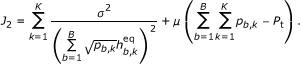(15)

Now, setting the partial derivatives of J2 to zero and after some mathematical manipulations, the powers p b,k can be shown to be given by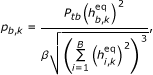(16)

where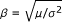. As for the first approach, Equation 16 can be re-written by replacing β by β b , b = 1, ..., B, which are computed to satisfy the individual per-BS power constraints and the closed-form solution achieved is then given by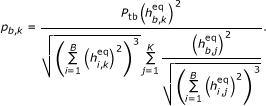(17)

This second suboptimal scheme is referred to as per-BS closed-form power allocation (per-BS SOCPA).

### Extension for OFDM systems

The previous results considering a single carrier can be easily generalized for an OFDM-based system, assuming N c parallel frequency flat fading channels. Two approaches can be considered: the above solutions are computed individually on each sub-carrier, i.e., the power per subcarrier is constrained to Ptb, or alternatively, the objective functions can be minimized jointly over all the available subcarriers, and the overall power of each BS is constrained to NcPtb. Clearly, the latter approach is more efficient, since we have more degrees of freedom to minimize the objective functions. For this case, the OPA problem can be formulated as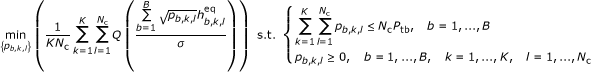(18)

where p b,k,l and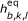are the allocated power and the equivalent frequency flat fading channel gain of the UT k on sub-carrier l and BS b, respectively. As in Equation 10, the objective function is convex in p b,k,l , and the constraint functions are linear; therefore, this is also a convex optimization problem, which can be solved using the interior-point method.

For the two suboptimal schemes, the powers can be obtained following the same two-phase approach as for the single carrier case. Thus, the solution for the joint subcarrier power allocation for per-BS SOIPA can be written as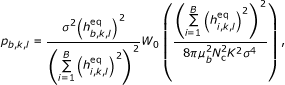(19)

and now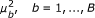are computed to satisfy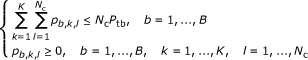.

Using the same approach, the solution that minimizes the sum of inverse of SNRs (per-BS SOCPA) for the joint subcarrier power allocation is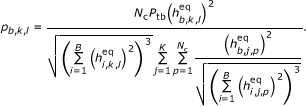(20)

The precoder vectors are designed by assuming that BSs have only knowledge of local CSI. However, since we consider a centralized power allocation, to compute all powers, the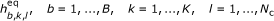coefficients should be available at the JPU. In our multicell system, each BS should send a real vector of size KNc to the JPU. It is noted that if the precoder vectors were computed in a centralized manner at the JPU, each BS should send to the JPU a complex vector of size NtbKNc, i.e., 2Ntb more information.

Although, in this study single antenna UTs were assumed, the formulation can be straightforwardly extended for multiple antenna UTs just by considering each antenna as a single antenna UT. The main difference is that the long-term channel power will be the same for all antennas belonging to the same UT.

### Numerical results

In order to evaluate the proposed solutions, we assume a typical pedestrian scenario based on LTE specifications . Two different scenarios are considered:

• Scenario 1, we assume that each supercell has two BSs, B = 2, which are equipped with two antennas, Ntb = 2, and two single antenna UTs, K = 2.

• Scenario 2, we assume that each supercell has four BSs, B = 4, which are equipped with four antennas, Ntb = 4, and four single antenna UTs, K = 4.

For both scenarios, the main parameters used in the simulations are, FFT size of 1024; number of resources, i.e., available subcarriers (Nc) shared by the K users set to 16; sampling frequency set to 15.36 MHz; useful symbol duration is 66.6 μs; cyclic prefix duration is 5.21 μs; overall OFDM symbol duration is 71.86 μs; subcarrier separation is 15 kHz; and modulation is 4-QAM.

We used the ITU pedestrian channel model B , with the modified taps' delays according to the sampling frequency defined on LTE standard. Concerning the MISO model, two approaches are considered: in the first one, we assume that the distance between antenna elements of each BS is far apart to assume uncorrelated channels, i.e., R = INtb. In the second, the average angle of departure (AoD) set to 60°, standard deviation of AoD set to 40°, and antenna spacing in all BSs set to half of wavelength. We use the same spatial transmitter correlation matrix for each BS, and also to simplify the channel model, we used the same correlation matrix for all the taps. We assume that each UT is placed on each cell. The long-term channel powers are assumed to be ρ b,k = 1, b = k for the intracell links, and ρ b,k , bk are uniformly distributed on the interval [0.2, 0.6] for the intercell links.

We compare the performance results of four precoding schemes with different per-BS power allocation approaches: per-BS equal power allocation (per-BS EPA), in this case p b,k,l = Ptb/K, (b,k,l); the two suboptimal approaches per-BS SOIPA and per-BS SOCPA and the optimal one per-BS OPA. We present results for two different approaches: for the case where the power is constrained per-subcarrier, i.e., the power per-subcarrier is fixed to Ptb but may vary from user to user, these curves are referred to as per-subcarrier power constraint, Per subcarrier PC. In this approach, the powers of each user are computed individually on each subcarrier. In the second one, the powers are computed jointly for all the available subcarriers, i.e., the overall power is fixed to PtbNc and may vary from subcarrier to subcarrier and from user to user. These curves are referred as joint subcarrier power constraint, Joint subcarrier PC. Also, we present results for optimal approach considering total power allocation (TPC OPA), as formulated in Equation 11. This is similar to the single cell scenario where the powers are computed to satisfy the overall power constraint, i.e.,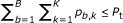, and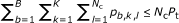if the powers are computed individually per subcarrier and jointly for all subcarriers, respectively. This serves as lower bound for the multicell scenario under per-BS power constraint. All the results are presented in terms of the average BER as a function of per-BS SNR defined as SNR = Ptb / ∑2.

Figure 3 shows the performance results of all the considered precoding schemes for scenario 1 and uncorrelated channels, i.e., R = INtb. It can be observed that the per-BS SOCPA, per-BS SOIPA, and per-BS OPA schemes outperform the per-BS EPA approach, because they redistribute the powers across the different sub-channels more efficiently. Considering a Per subcarrier PC strategy, the performance of the two proposed suboptimal per-BS approaches is very close. Moreover, the performance penalty of the two suboptimal schemes against the optimal one is low, less than 0.2 dB for a BER = 10-3. Also, the penalty of the per-BS OPA against the lower bound given by the TPC OPA is approximately 0.3 dB considering also a BER = 10-3. The results show that the proposed precoding schemes with Joint subcarrier PC clearly outperform the same ones with Per subcarrier PC. For this case, the performance of the suboptimal per-BS SOIPA and optimal per-BS OPA is also very close (penalty less than 0.1 dB), but the gap between these two schemes and the suboptimal per-BS SOCPA increases for the Joint subcarrier PC approach. These results show that the per-BS SOIPA only outperforms the per-BS SOCPA for a large number of sub-channels. We can observe a penalty of approximately 0.6 dB of the per-BS SOCPA scheme against the per-BS SOIPA for a BER = 10-3. Also, a gain of approximately 1.2 and 4.2 dB of the suboptimal per-BS SOIPA scheme against the per-BS EPA is obtained (BER = 10-3) for Per subcarrier PC and Joint subcarrier PC approaches, respectively.

The simulations leading to Figure 4 were obtained for scenario 2 and uncorrelated channels. Comparing the results obtained for this scenario with the ones obtained for scenario 1, we can observe a considerable gain. This is because now each UT receives the same data from four different BSs instead of only two, increasing the diversity order and also the antenna array gain since the different copies are coherently combined in the receiver. We also can see here that all proposed schemes outperform the per-BS EPA. Considering a Per subcarrier PC strategy, in this scenario, the performance of the two proposed suboptimal per-BS approaches is very close. Moreover, the performance penalty of the two suboptimal schemes against the optimal one is low, less than 0.5 dB for a BER = 10-3. Also, the penalty of the per-BS OPA against the lower bound given by the TPC OPA is higher than in the first scenario and is approximately 0.5 dB considering also a BER = 10-3. This is because in this scenario the number of power constraints is higher than the previous one since the number of BSs is increased to four. The results also show that the proposed precoding schemes with Joint subcarrier PC clearly outperform the same schemes with Per subcarrier PC for the same reasons explained for scenario 1. Another important issue that should be emphasized is that the penalty of the per-BS OPA against the TPC OPA is approximately of 0.5 dB for the Per subcarrier PC, but is reduced to less than 0.1 dB with Joint subcarrier PC (BER = 10-3), because the number of degrees of freedom to minimize the average BER is increased. Intuitively, the penalty decreases as the number of sub-channels increases, i.e., the performance of the per-BS OPA tends to the performance of the TPC OPA when the number of sub-channels tends to infinity.

In Figures 5 and 6, we present the performance results for the same precoding schemes, but considering scenarios 1 and 2 with correlated channels, respectively. From these figures, we basically can arrive at the same conclusions as for the results obtained in the previous ones. However, a penalty of approximately 3 and 4 dB can be observed in all curves, assuming a BER = 10-3, as compared with the ones presented in Figures 3 and 4, respectively. As discussed in section "System model",are Gaussian random variables with variance δ b,k ρ b,k , (b,k) per component, where ρ b,k is the variance considering R = INtb, and hence, a performance penalty is obtained as compared with uncorrelated channel antenna scenarios.

## Conclusions

We proposed and evaluated in this study power allocation schemes for the downlink of distributed precoded multicell-based systems. The criteria considered were the minimization of the BER and two centralized power allocation algorithms with per-BS power constraint: one optimal that can be achieved at the expense of some complexity and one suboptimal with lower complexity aiming at practical implementations. Both in the optimal (per-BS OPA) and in the suboptimal (per-BS SOIPA), the computation of the transmitted powers required an iterative approach. To circumvent the need for iterations, we further proposed another suboptimal scheme (per-BS SOCPA), where the power allocation was computed to minimize the sum of inverse of SNRs of each UT allowing us to achieve a closed-form solution.

The results have shown that the proposed multiuser multicell schemes cause significant improvement in system performance, in comparison with the equal power allocation approach, namely, for the case of Joint subcarrier PC. Also, the performance of the proposed suboptimal algorithms, namely, the per-BS SOIPA approach, is very close to the optimal with the advantage of lower complexity. Moreover, when comparing the iterative and closed form schemes, results have shown that the per-BS SOIPA outperforms the per-BS SOCPA only when Joint subcarrier PC strategy is considered. Another important conclusion is that the penalty performances of the per-BS SOIPA and per-BS OPA schemes are negligible against the lower bound TPC OPA, mainly for large number of joint processing sub-channels.

The results allow us to conclude that the suboptimal proposed power allocation schemes allow for a significant performance improvement with very low UT and moderate complexities both at the BS and JPU, and therefore, present significant interest for application in the next generation wireless networks for which cooperation between BSs is anticipated.

## Appendix

In this appendix, we prove that the solution that minimizes Equation 11 is given by Equation 13. As stated above, the powers p b,k , b = 1, ..., B, k = 1, ... K can be determined by setting the partial derivatives of J_1 to zero, obtaining the following set of equations: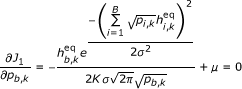(21)

Since we have the same Lagrange multiplier, μ, in all BK equations, it is possible to establish the following power relations: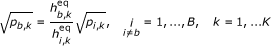(22)

Using Equation 22 in 21 we have,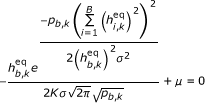(23)

Then, both terms of Equation 23 are squared: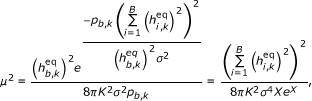(24)

where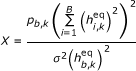. Then, the problem reduces to solving an exponential equation of type XeX= a, with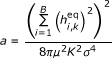, and the solution can be given by the Lambert function of index 0 :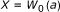(25)

Finally, replacing x and a in Equation 25, we obtain the powers that minimize Equation 11(26)

## End notes

aHere, K is the number of users that share the same set of resources. Considering an OFDMA-based system, the total number of users can be significantly larger than K, since different set of resources can be shared by different set of users

## Abbreviations

AoD:

angle of departure

BD:

block diagonalization

BER:

bit error rate

CSI:

channel state information

CU:

central unit

DPC:

dirty paper coding

JPU:

joint processing unit

OFDM:

orthogonal frequency division multiplexing

TPC:

total power constraint

UTs:

user terminals

ZF:

zero forcing.

## References

1. Foschini GJ, Gans MJ: On limits of wireless communications in a fading environment when using multiple antenna. Wirel. Personal Commun. Mag 1998, 6(3):311-335. 10.1023/A:1008889222784

2. Uppala LS, Li J: Designing a mobile broadband wireless access network. IEEE Signal Process. Mag 2004, 21(5):20-28. 10.1109/MSP.2004.1328085

3. 3GPP Long Term Evolution[http://www.3gpp.org/LTE]

4. Andrews JG, Choi W, Heath RW: Overcoming interference in spatial multiplexing MIMO cellular networks. IEEE Wirel. Commun. Mag 2007, 47(6):95-104.

5. Karakayali M, Foschini G, Valenzuela R: Network coordination for spectral efficient communications in cellular systems. IEEE Wirel. Commun. Mag 2006, 13(4):56-61. 10.1109/MWC.2006.1678166

6. Mudumbai TR, Brown DR, Madhow U, Poor HV: Distributed transmit beamforming: challenges and recent progress. IEEE Commun. Mag 2009, 47(2):102-110.

7. European ICT FUTON project[http://www.ict-futon.eu]

8. Diehm F, Marsch P, Fettweis G: The FUTON prototype: proof of concept for coordinated multi-point in conjunction with a novel integrated wireless/optical architecture. Proc. IEEE WCNC'10 2010.

9. Jing S, Tse DNC, Sorianga JB, Hou J, Smee JE, Padovani R: Multicell downlink capacity with coordinated processing. EURASIP J. Wirel. Commun. Netw 2008, 2008(2008):1-19.

10. Somekh O, Zaidel B, Shamai S: Sum rate characterization of joint multiple cell-site processing. IEEE Trans. Inf. Theory 2007, 53(12):4473-4497.

11. Boccardi F, Huang H: Limited downlink network coordination in cellular networks. Proc. IEEE PIMRC'07 2007.

12. Zhang J, Chen R, Andrews JG, Heath RW Jr: Coordinated multicell MIMO systems with cellular block diagonalization. Proc. IEEE ACSSC'07 2007, 1669-1673.

13. Zhang J, Chen R, Andrews JG, Ghosh A, Heath RW Jr: Networked MIMO with clustered linear precoding. IEEE Trans. Wirel. Commun 2009, 8(4):1910-1921.

14. Marsch P, Fettweis G: On downlink network MIMO under a constrained backhaul and imperfect channel knowledge. Proc. IEEE GLOBECOM'09 2009.

15. Armada AG, Fernándes MS, Corvaja R: Waterfilling schemes for zero-forcing coordinated base station transmissions. Proc. IEEE GLOBECOM'09 2009.

16. Kobayashi M, Debbah M, Belfiore J: Outage efficient strategies in network MIMO with partial CSIT. Proc. IEEE ISIT'09 2009, 249-253.

17. Papadogiannis A, Hardouin E, Gesbert D: Decentralising multicell cooperative processing: a novel robust framework. EURASIP J. Wirel. Commun. Netw 2009, 2009(2009):1-10.

18. Zhang R: Cooperative multi-cell block diagonalization with per-base-station power constraints. Proc. IEEE WCNC'10 2010.

19. Bjornson E, Zakhour R, Gesbert D, Ottersten B: Cooperative multicell precoding: rate region characterization and distributed strategies with instantaneous and statistical CSI. IEEE Trans. Signal Process 2010, 58(8):4298-4310.

20. Costa MHM: Writing on dirty paper. IEEE Trans. Inf. Theory 1983, 29(3):439-441. 10.1109/TIT.1983.1056659

21. Bengtsson YK, Ottersten M, McNamara B, Karlsson D, Beach P: Modeling of wide-band MIMO radio channels based on NLoS indoor measurements. IEEE Trans. Veh. Technol 2004, 3(3):655-665.

22. Zhao J, Kuhn M, Wittneben A: Cooperative transmission schemes for decode-and-forward relaying. Proc. IEEE PIMRC'07 2007.

23. Lozano A, Tulino AM, Verdú S: Optimum power allocation for multiuser OFDM with arbitrary signal constellations. IEEE Trans. Commun 2008, 56(5):828-837.

24. Proakis J: Digital Communications. 3rd edition. McGraw-Hill, New York; 1995.

25. Boyd S, Vandenberghe L: Convex Optimization. Cambridge University Press, Cambridge; 2004.

26. Haykin S: Adaptive Filter Theory. 3rd edition. Prentice Hall; 1996.

27. Corless RM, Gonnet GH, Hare DEG, Jeffrey DJ, Knuth DE: On the Lambert W function. Adv. Comput. Math 1996, 5: 329-359. 10.1007/BF02124750

28. Rostaing P, Berder O, Burel G, Collin L: Minimum BER diagonal precoder for MIMO digital transmissions. Elsevier Signal Process 2002, 82(10):1477-1480.

29. Palomar DP, Cioffi JM, Lagunas MA: Joint Tx-Rx beamforming design for multicarrier MIMO channels: a unified framework for convex optimization. IEEE Trans. Signal Process 2003, 51(9):2381-2401. 10.1109/TSP.2003.815393

30. 3rd Generation Partnership Project, "LTE Physical Layer--General Description 2007.

31. Guidelines for the evaluation of radio transmission technologies for IMT-2000 1997.

## Acknowledgements

This study presented in this paper was supported by the European FUTON project, FP7 ICT-2007-215533 and the Portuguese CADWIN project, PTDC/EEA TEL/099241/2008.

## Author information

Authors

### Competing interests

The authors declare that they have no competing interests

## Authors’ original submitted files for images

Below are the links to the authors’ original submitted files for images.

## Rights and permissions

Reprints and Permissions

Silva, A., Holakouei, R. & Gameiro, A. Power allocation strategies for distributed precoded multicell based systems. J Wireless Com Network 2011, 1 (2011). https://doi.org/10.1186/1687-1499-2011-1

• Accepted:

• Published:

• DOI: https://doi.org/10.1186/1687-1499-2011-1

### Keywords

• Cooperative systems
• multicell precoding
• power allocation
• bit error rate
• orthogonal frequency division multiplexing (OFDM)